Which of the following figure has six faces?

# Which of the following figure has six faces?

1. A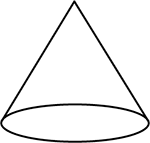2. B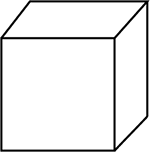3. C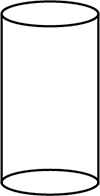4. D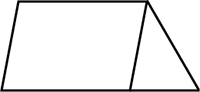Fill Out the Form for Expert Academic Guidance!l

+91

Live ClassesBooksTest SeriesSelf Learning

Verify OTP Code (required)

### Solution:

It is given a set of figures.
We have to find the figure which has 6 faces.
In option 1, we have cone.Here, it has only 2 faces.
In option 2, we have a cube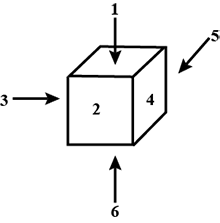Here, it has 6 faces.
In option 3, we have cylinder.Here, it has only 3 faces.
In option 4, we have a 2-D shapeHere, since the given structure is 2-D shape, it does not have any face.
Therefore, a cube with six faces.
Hence, the correct option is 2.

## Related content

 Area of Square Area of Isosceles Triangle Pythagoras Theorem Triangle Formula Perimeter of Triangle Formula Area Formulae Volume of Cone Formula Matrices and Determinants_mathematics Critical Points Solved Examples Type of relations_mathematics+91

Live ClassesBooksTest SeriesSelf Learning

Verify OTP Code (required)